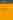# Linear Processes in Function Spaces. - Theory and Applications

## Denis Bosq

Note moyenneThe main subject of this book is the estimation and forecasting of continuous time processes. It leads to the development of the theory of linear processes... Lire la suite
44,60 € Neuf

## Résumé

The main subject of this book is the estimation and forecasting of continuous time processes. It leads to the development of the theory of linear processes in function spaces. The necessary mathematical tools are presented in Chapters 1 and 2. Chapters 3 to 6 deal with autoregressive processes in Hilbert and Banach spaces. Chapter 7 is devoted to general linear processes and Chapter 8 to statistical prediction. Implementation and numerical applications appear in Chapter 9. The book assumes a knowledge of classical probability theory and statistics.

## Sommaire

• Stochastic processes and random variables in function spaces
• Sequences of random variables in Banach spaces
• Autoregressive Hilbertian processes of order one
• Estimation of autocovariance operators for ARH(1) processes
• Autoregressive Hilbertian processes of order p
• Autoregressive processes in Banach spaces
• General linear processes in function spaces
• Estimation of autocorrelation operator and prediction
• Implementation of functional autoregressive predictors and numerical applications

## Caractéristiques

• Date de parution
06/09/2000
• Editeur
• Collection
• ISBN
0-387-95052-4
• EAN
9780387950525
• Présentation
Broché
• Nb. de pages
283 pages
• Poids
0.445 Kg
• Dimensions
15,5 cm × 23,5 cm × 1,7 cm

## À propos de l'auteur

### Biographie de Denis Bosq

D. Bosq is Professor of Statistics at the University of Paris VI (Pierre et Marie Curie). He is Editor-in-Chief of Statistical Inference for Stochastic Processes and of Annales de l'ISUP, and Associate Editor of the Journal of Nonparametric Statistics. He is an elected member of the International Statistical Institute. He has published about 100 papers or works on nonparametric statistics and five books including Nonparametric Statistics for Stochastic Processes: Estimation and Prediction, Second Edition (Springer, 1998).

44,60 €